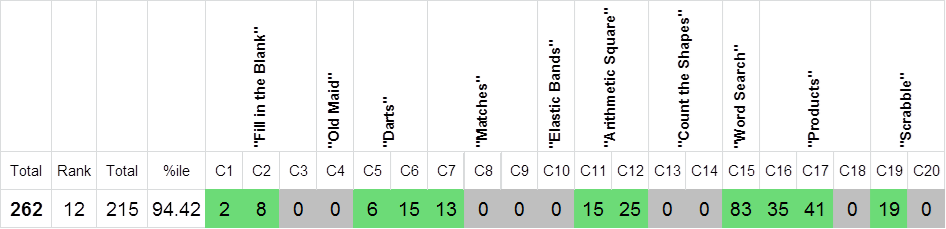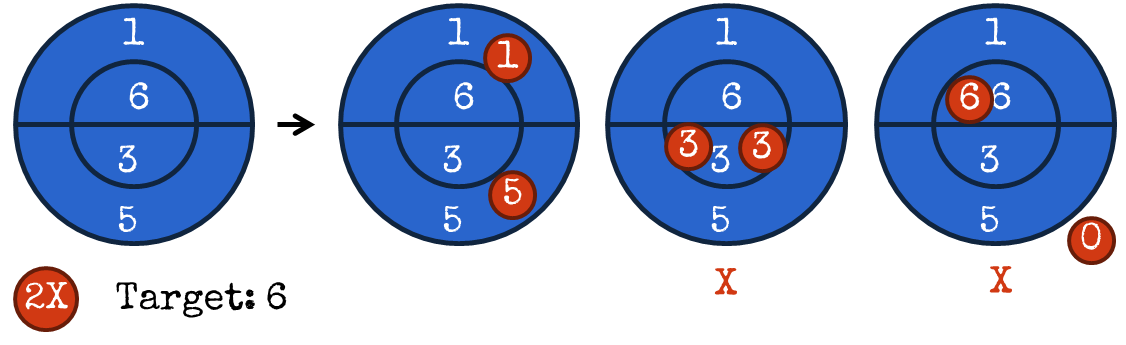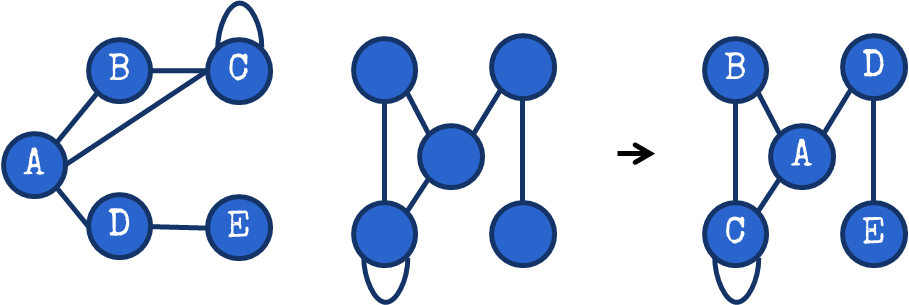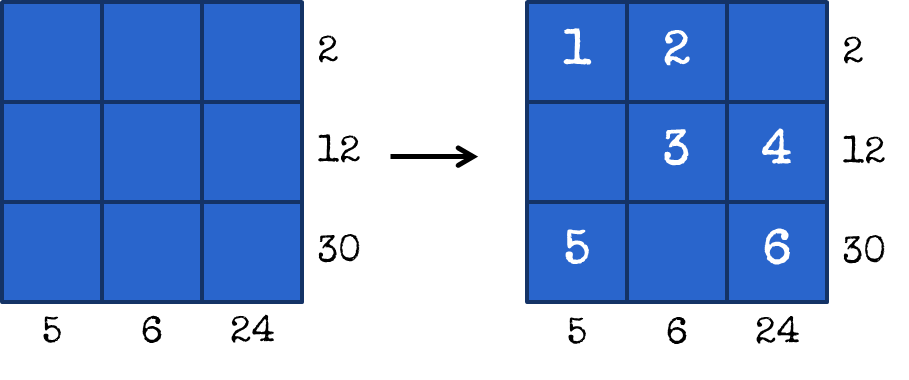# Unwinding Logical Threads (Hotseat: WPF Puzzle GP 2017, Round 1C)#### Background

The World Puzzle Federation is an international organisation which, among other things, organises annual puzzle contests. These involve solving puzzles that rely on logical deduction and observation. That said, maybe because I’m pretty new to these, sometimes backtracking algorithms are the order of the day!

The Puzzle GP is a series of distributed puzzle competitions run throughout the year. The GP is split into three series: C, B and A (in increasing order of difficulty). Puzzles in group C are likely to be largely accessible to general audiences (and that’s what I’m covering today); group B puzzles are typically drawn from a selection of “well-known” puzzle types, and group A puzzles often feature extensions of group B puzzles in unusual ways.

Contestants are given 60 minutes for each set of puzzles. I have very little experience with these puzzles, so I think I’ll start with group C.

(The puzzle set can be found here.)

#### PerformanceI started off with the Darts puzzles, for which I had a strategy in mind. I then struggled with the Matches puzzles, only solving C7, before moving on to the Arithmetic Squares, both of which were solved quickly. I then spent probably a good ten minutes digging through the Word Search. I finished the easy Scrabble task and almost finished the harder one, though I failed to see a simple move near the end of the puzzle. I then started on Products, which I probably should have prioritised more highly as I think I figured out the mathematical logic behind it. I solved the 120-pointer C18 after the contest, and it was actually not too hard. With three minutes left to go after finishing C17, I took a stab at the Fill in the Blank series and managed to solve two of them.

I solved the remaining puzzles after the time ran out, apart from C3. Elastic Bands was actually very easy. I have a bit of an aversion to spatial reasoning puzzles, but recognised the problem as graph isomorphism and had some techniques for it based on that. Old Maid was also easy; I didn’t get round to it in time. The last Fill in the Blank and both instances of Count the Shapes both gave me headaches.

#### Selected Problems in Depth

##### C5-C6: DartsGiven a dartboard which has several regions with$k$ point values and$m$ darts, find a way to throw the darts on the dartboard such that the darts add up to a target value. Each dart must score, and each region can have only up to 1 dart. For example, with regions$[4, 21, 8, 11, 13]$, two darts and a target of$19$, the correct answer is$[8, 11]$.

To solve this for two darts, one good solution could be to iterate through the list. On a computer, using a hash-set could be a good solution; when we look at a number, if it’s in our “target set” we’re done – otherwise we add the number we would need to make up the target to the target set. It’s a simple$O(n)$ solution.

I think implementing a hash set on paper for me would be too troublesome. There’s an alternative which I used: sort the array in$O(n \log n)$ and then, use a pair of pointers, one starting at the beginning and one at the end of the array. Adding the numbers up, if we’re over the target we bring the pointer at the end back, and if we’re under the target we push the pointer at the start forward.

The problem had three darts; I simply picked the overall largest number and solved the appropriately reduced target with the two-dart algorithm above, backtracking where needed to smaller largest numbers. Binary insertion sort is probably pretty reasonable to do by hand.

##### C10: Elastic BandsGiven a network with nodes marked with letters, and another network with edges provided, give a labelling of the nodes such that the networks are identical. This is basically finding what’s called a graph isomorphism in discrete mathematics.

I didn’t solve this in the contest, but probably should have. I split the nodes by degree, and then tried to identify relationships between these. For example, there was a single degree 4 node in the puzzle that wasn’t connected to any of the other degree 4 nodes, so I was able to uniquely identify it quickly.

##### C16-C18: ProductsGiven an$N \times N$ grid, place the numbers from$1, 2, \ldots, 2N$ in the grid once each. Each row and column must contain two numbers, and numbers cannot share a cell. Clue numbers are given by the side of the grid and sometimes on diagonals. Where given, the product of numbers in the relevant row, column or diagonal must equal the clue.

The first two puzzles had$N=10$; the third had$N=15$. I think my main strategy here involved searching for prime factors that would uniquely identify numbers along the rows and columns. For$N=10$ this would be$[11, 13, 17, 19]$; for$N=15$ this included$[17, 19, 23, 29]$. I also usually started looking for very large numbers (because they might have a unique factorization in the allowed range) or small ones (e.g. in the first puzzle, there was only one column and one row with a product less than or equal to 20, implying that the 1 had to be in that column/row).

I also considered the places where a given number might fit, and in the later puzzles noticed when multiple columns could definitively use certain numbers. For example, if 5 was already used in an$N=10$ puzzle, and there were two 20 row hints, I could eliminate 1, 20, 2 and 10 from consideration for the other rows.

This wasn’t too difficult, though I can imagine that puzzles with only partial hints might be substantially more challenging!

#### Meta-Analysis

A score of 262 would have placed 12th out of 215 contestants (2nd out of 139 for contestants not in higher divisions). To be fair, this is in part because many top solvers don’t participate in the division C contests at all. Some of this might also be because there would have been less pressure as I was doing this on my own, not as part of a real contest.

Looking at the top end of the scoreboard, the Darts, Arithmetic Square, first instance of Matches and first instance of Products were solved by most of the leaders. Elastic Bands and Old Maid (I didn’t solve either of these two), as well as the Word Search were also fairly common. Thereafter, contestants varied quite a bit in what they solved. As might be expected, the contestants that solved the final instance of Products generally did very well (it was worth 120 points by itself).